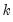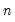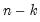makepcomp
Save the scores from a principal components analysis of the series in a group.
Syntax
group_name.makepcomp(options) output_list
where the output_list is a list of names identifying the saved components. EViews will save the firstcomponents corresponding to theelements in output_list, up to the total number of series in the group.
Options

 scale=arg (default=“normload”) Diagonal matrix scaling of the loadings and the scores: normalize loadings (“normload”), normalize scores (“normscores”), symmetric weighting (“symmetric”), user-specified (arg=number). cpnorm Compute the normalization for the score so that cross-products match the target (by default, EViews chooses a normalization scale so that the moments of the scores match the target). eigval=vec_name Specify name of vector to hold the saved the eigenvalues in workfile. eigvec=mat_name Specify name of matrix to hold the save the eigenvectors in workfile. prompt Force the dialog to appear from within a program.
Covariance Options

 cov=arg (default=“corr”) Covariance calculation method: ordinary (Pearson product moment) covariance (“cov”), ordinary correlation (“corr”), Spearman rank covariance (“rcov”), Spearman rank correlation (“rcorr”), uncentered ordinary correlation (“ucorr”). Note that Kendall’s tau measures are not valid methods. wgt=name (optional) Name of series containing weights. wgtmethod=arg (default = “sstdev” Weighting method: frequency (“freq”), inverse of variances (“var”), inverse of standard deviation (“stdev”), scaled inverse of variances (“svar”), scaled inverse of standard deviations (“sstdev”).Only applicable for ordinary (Pearson) calculations where “weights=” is specified. Weights for rank correlation and Kendall’s tau calculations are always frequency weights. pairwise Compute using pairwise deletion of observations with missing cases (pairwise samples). partial=arg Compute partial covariances conditioning on the list of series specified in arg. df Compute covariances with a degree-of-freedom correction accounting for the mean (for centered specifications) and any partial conditioning variables. The default behavior in these cases is to perform no adjustment (e.g. – compute sample covariance dividing byrather than).
Examples
grp1.makepcomp comp1 comp2 comp3
saves the first three principal components (in normalized loadings form) to the workfile. The components will have variances that are proportional to the eigenvalues.
grp1.makepcomp(scale=normscore) comp1 comp2 comp3
normalizes the scores so that the resulting series have variances that are equal to 1.
You may change the scaling for the normalized components so that the cross-products equal 1, using the cpnorm option:
grp1.makepcomp(scale=normscore, cpnorm) comp1 comp2 comp3
Cross-references
See “Saving Component Scores” for further discussion.
See Group::pcomp for tools to display the principal components results for the series in the group.